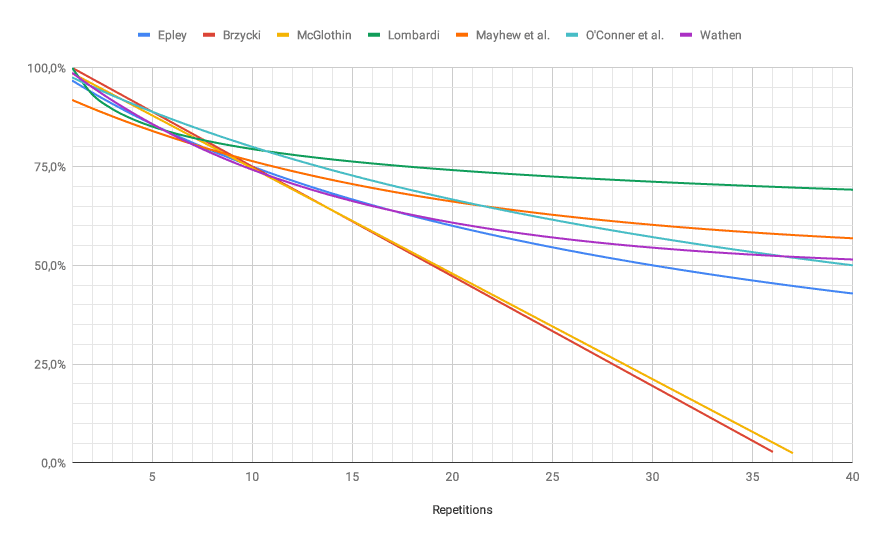# 1rm Calculator

Enter Information

#### RESULTS

Fill the calculator form and click on Calculate button to get result here

## What is One Rep Max?

1RM stands for one rep maximum. The overall weight that can be lifted in an attempt is one rep max. To measure the real strength, it could be the best way out there.

The most precise method to check the one rep max is to use a barbell for testing. Nonetheless, this can affect the plan, recovery time, and theoretically increase the likelihood of injury.

Different methods are used to calculate 1RM. Brzycki, Epley, and Landers established formulas that are very reliable and common. More experienced lifters may need to check NSCA (National Strength and Conditioning Association) figures for exercise.

## 1rm formula

You can estimate your 1RM with various formulas. These formulas are slightly different in calculations. The Brzycki formula from Matt Brzycki is the most popular and tested for accuracy.

1rm = weight / ( 1.0278 – 0.0278 × reps )

### One Rep Max calculation Example

If you could lift 80 kg for 5 repetitions, the 1RM would be calculated as:

1rm = $$\dfrac{80}{( 1.0278 - 0.0278 \times 5 )} = 90 kg$$

If your program is like this, you can continue to lift your five reps while still gaining an idea of your strength.

1RM estimation will be more unreliable if you are further away from one rep. Say that you can lift 10 kg to 30 reps, your 1RM would be 52 kg with Brzycki's formula. Nonetheless, the trust of this number is so poor that the estimate is not worthwhile.

As a guideline, don't think about anything more than 10 reps. You may know that you usually get 5 kg of the real 1RM, or more or less for planning purposes.

You can measure the 1RM for more than a single way. These are the different formulas to calculate your 1RM:

### Brzycki Formula

1rm = $$\text{weight} \times \Big(\dfrac{36 }{(37 - \text{reps})}\Big)$$

### Epley Formula

1rm = $$\text{weight} \times (1 + 0.0333 \times \text{reps})$$

### Lander Formula

1rm = $$\dfrac{(100 \times \text{weight})}{(101.3 - 2.67123 \times \text{reps})}$$

### Lombardi Formula

1rm = $$\text{weight} \times \text{reps}$$

### Mayhew et al. Formula

1rm = $$\dfrac{(100 \times \text{weight})}{(52.2 + (41.9 \times e^{-0.055} \times \text{reps}))}$$

### O’Conner et al. Formula

1rm = $$\text{weight} \times (1 + 0.025 \times \text{reps})$$

### Wathan Formula

1rm = $$\dfrac{(100 \times \text{weight})}{(48.8 + (53.8 \times e^{-0.075} \times reps))}$$

## 1 Rep Max ChartBy WheyProteinIsolate - Own work, CC BY-SA 4.0, Link

## How to calculate one rep max?

You may assume that your 1RM limit doesn't matter because you won't practice the heavy lifts anyway. One day, however, you may see a program demanding 65 percent of your 1RM to be used on a lift. In this scenario, you either have to check or measure the 1RM.

Testing the 1RM can be very painful, and a high degree of vigilance is needed to prevent injuries. The good news is that you can calculate your 1RM without taking a test. Exercise researchers created a method that is pretty precise to calculate for most people and this one (1) rep max calculator is based on the same formula.

example

Let's assume you can raise 220 pounds on the bent-over row for ten reps, with good form. This helps us not only to measure the one-rep max, but also other loads, which include your 70% 1RM, 55% 1RM, or 85% 1RM, depending on the requirements of your program.

The formula calculates the 1rm using the total weight you can raise during a move given to you and the number of reps that you can do before tiredness or slowing down. Let's make sure you can make 220 pounds for 10 reps for the chain. Put these values in the above calculator, and you will see the estimated 1rm.

## 1 REP-MAX Percentages Table

The following 1 rep max chart represents the percentage of 1rm against the number of reps.

RepetitionsPercentage of 1RM
1100%
297%
394 %
492%
589%
686%
783%
881%
978%
1075%
1173%
1271%
1370%
1468%
1567%
1665%
1764%
1863%
1961%
2060%
2159%
2258%
2357%
2456%
2555%
2654%
2753%
2852%
2951%
3050%

Wikimedia Foundation. (2021, November 26). One-repetition maximum. Retrieved December 13, 2021
How to calculate your One rep max, explained by a strength and conditioning coach. Menshealth.com. Retrieved December 13, 2021.
Other Languages
User Ratings
• Total Reviews 0
• Overall Rating 0/5
• Stars
Reviews
No Review Yet